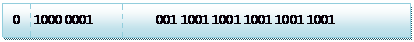﻿ 【转】浮点数在计算机中的存储方式

### 【转】浮点数在计算机中的存储方式

C语言和 C#语言中，对于浮点型的数据采用单精度类型(float)和双精度类型(double)来存储：

float 数据占用 32bit；

double 数据占用 64bit；

float 遵从的是 IEEE R32.24；

double 遵从的是 IEEE R64.53；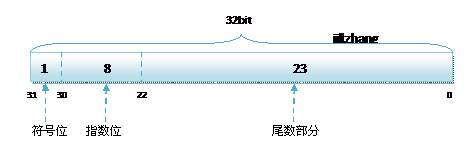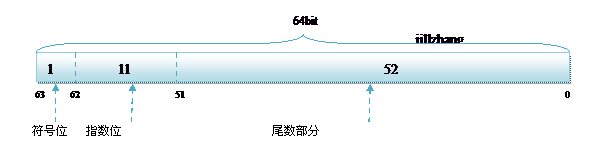R32.24 和 R64.53 的存储方式都是用科学计数法来存储数据的，比如：

8.25  用十进制表示为：8.25 * 100

120.5 用十进制表示为：1.205 * 102

8.25   用二进制表示为：1000.01

118.5 用二进制表示为：1110110.1

8.25 用二进制表示为：1000.01

8.25 用二进制的科学计数法表示为: 1.0001* 2，按照上面的存储方式：

符号位为：0，表示为正；

指数位为：3+127=130，即 10000011；

尾数部分为：0001；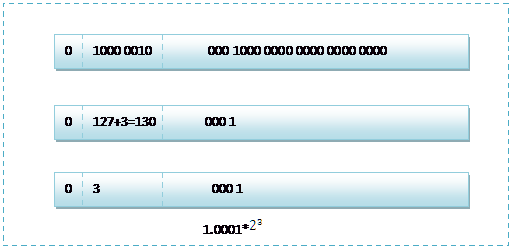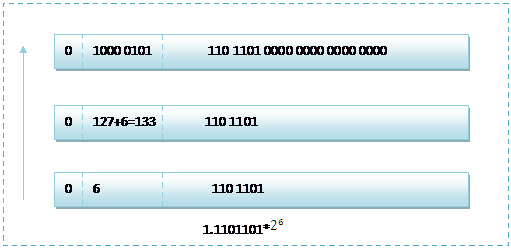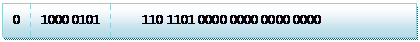1.1101101*2(133-127=6) = 1.1101101 * 2= 1110110.1=118.5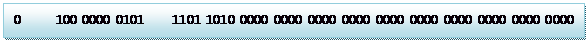```class 浮点数
{
static void Main(string[] args)
{
float f = 2.2f;
double d = (double)f;
Console.WriteLine(d.ToString("0.0000000000000"));
//结果："2.2000000476837"

f = 2.25f;
d = (double)f;
Console.WriteLine(d.ToString("0.0000000000000"));
//结果："2.2500000000000"

//2.25 - 2.2 = 0.05 ( 但实际结果不是0.05 )
float f2 = 2.25f - 2.2f;
Console.WriteLine(f2.ToString("0.0000000000000"));
//结果："0.0499999500000"
}
}```

2.25 的单精度存储方式表示为：0 10000001 00100000000000000000000

2.25 的双精度存储方式表示为：0 10000000 0010010000000000000000000000000000000000000000000000000

0.2×2=0.4，所以二进制小数第一位为0.4的整数部分0；

0.4×2=0.8，第二位为0.8的整数部分0；

0.8×2=1.6，第三位为1；

0.6×2=1.2，第四位为1；

0.2×2=0.4，第五位为0；

...... 这样永远也不可能乘到=1.0，得到的二进制是一个无限循环的排列 00110011001100110011...